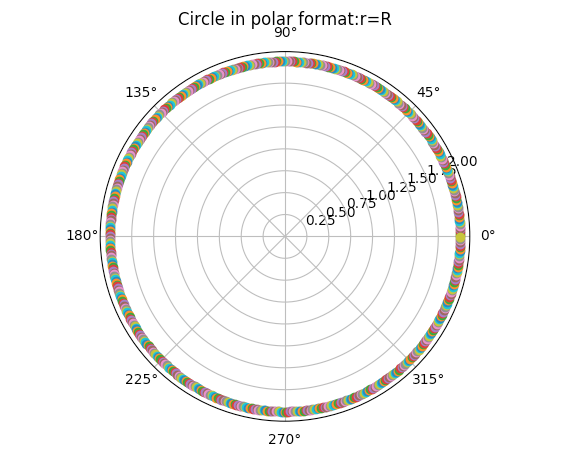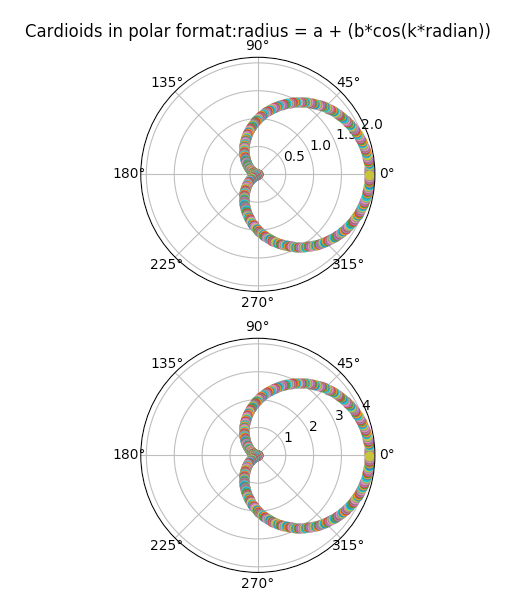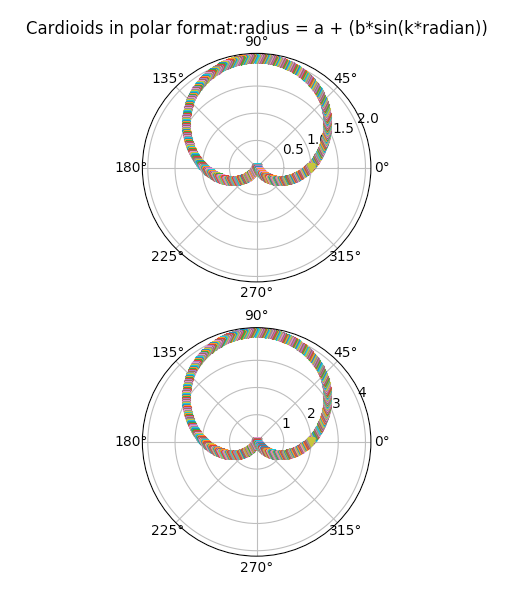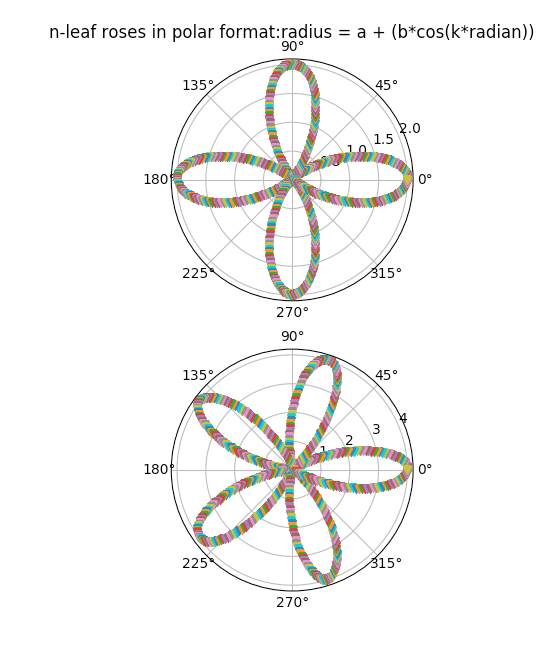# Polar Plots Using Python And Matplotlib

## Overview:

• A point in polar co-ordinates is represented as (r,Θ).

• The pair has a distance and angle – r for radius or distance from the origin and theta - Θ for the angle at which r has to be measured from origin.

• Polar co-ordinates and their modified counterparts are used in navigation, in describing the directionality of a microphone and in any system that is based on a central-point or origin.

• The pyplot module of Python Matplotlib provides the function polar which draws a polar plot.

• Remember, any mathematical function that can be plotted using the Cartesian coordinate system can be plotted using the polar co-ordinates as well.

## Example1- Circle:

The python code below plots a circle using polar form. The equation of the circle in polar form is given by r = R.

 # Example Python Program to plot a polar plot of a circle # import the numpy and pyplot modules import numpy as np import matplotlib.pyplot as plot plot.axes(projection='polar') # Set the title of the polar plot plot.title('Circle in polar format:r=R') # Plot a circle with radius 2 using polar form rads = np.arange(0, (2*np.pi), 0.01) for radian in rads:     plot.polar(radian,2,'o')  # Display the Polar plot plot.show()

## Output1-Circle:## Example2-Cardioids-Symmetrical around x-axis:

• When the radii of two circles are equal, and when one circle is fixed and the other circle is rolling on the first one – a point on the rolling circle traced will plot a cardioid.

• The polar forms of the cardioids are given by:

r = a + bcos(k*Θ)

r = a + bsin(k*Θ)

For the equation, r = a + bcos(k*Θ),

• When a=1, b=1 and k=1, the cardioids are symmetrical around x-axis on the positive side.

• When a=-1, b=-1 and k=1, the cardioids are symmetrical around x-axis on the negative side.

## Output2-Cardioids-Symmetrical around x-axis:## Example3-Cardioids-Symmetrical around y-axis:## Example4-n-leaf roses:

While keeping k > 1, and a=1,b=1,a=2,b=2 k-leaf roses are plotted.